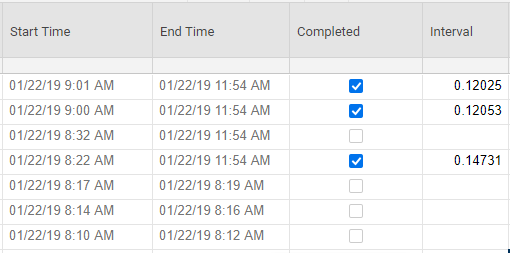# Determining duration in Hours:Minutes

Hey guys,

I have read several posts with several workarounds for calculating a value between a Start Time and an End Time where the input and result need to be in HH:MM. I have an idea and a beginning:

1. I created two System Columns. [Start Time] as 'Created (Date)' and [End Time] as 'Modified (Date).'

2. I created a [Completed] column as 'Checkbox.'

3 I created an [Interval] column to hold the time between [Start Time] and [End Time] which uses the simple formula:  =IF(Completed1 = "True", [End Time]1 - [Start Time]1)

This gives me raw time in "Smart Sheet Units" which seems to be: 1 Second = .0000116 SSU

If this is a feasible start, then I need to know if the following is possible.

4. Can I freeze or turn off the formula after the calculation is triggered by the checkbox so that the raw time is unaffected by further modification?

5. Convert the Raw time to Hours:Minutes?

6. Subtract non-work hours, weekends and holidays?

I am pretty sure I have seen you guys do everything but freeze a calculation. What do you think???

Thanks!

Kevin• Instead of converting the raw time, use something like this...

=INT((Modified@row - Created@row) * 24) + ":" + ROUND((((Modified@row - Created@row) * 24) - INT((Modified@row - Created@row) * 24)) * 60)

Unfortunately there is no way to freeze the Modified cell unless checking that box is the very last thing done on the row.

Working in part 6 of your original post is going to take some work and luck. I have an idea of how to make it happen, but its just a rough idea. I haven't worked out any of the formulas and details just yet. I'll have to get back to you on that part.

## Help Article Resources

Want to practice working with formulas directly in Smartsheet?

Check out the Formula Handbook template!